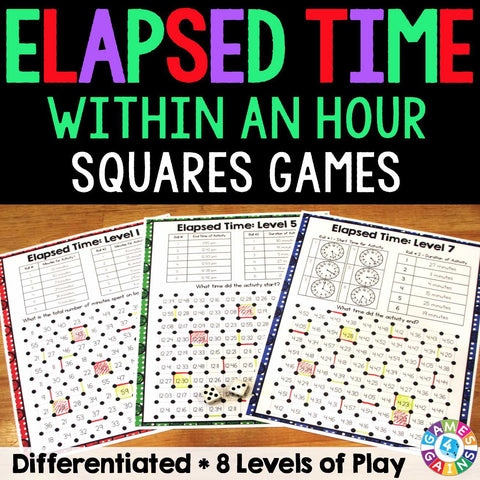## Elapsed Time Within An Hour "Squares" Game

• \$350

Elapsed Time Within An Hour "Squares" game contains 8 fun and engaging elapsed time games to help students practice determining concepts related to elapsed time.

In these games, students will practice:
• Subtracting minutes within 60
• Determining the amount of time elapsed when given start and end times (with digital and analog times)
• Determining the start time when given elapsed time and end time (with digital and analog times)
• Determining the end time (with digital and analog times)

Each one-page elapsed time game gets students to solve for the amount of time that has elapsed, the start time, or the end time. This means that you can have each student practicing the skill that he/she needs the most help with.

These Elapsed Time Within An Hour "Squares" games are so simple to use, and take a minimal amount of prep. Simply print out the game sheet, grab 2 dice and a few markers, and you'll be ready to go!

To play, students will roll the dice to find the time and solve the question on the game board. Then, students draw a line between two of the dots surrounding the answer. When a student's line forms a complete square, he/she gets to capture that square. The student with the most squares at the end of the game is the winner!

Included with this Elapsed Time Within An Hour "Squares" Game:
Instructions for play
8 "squares" game boards